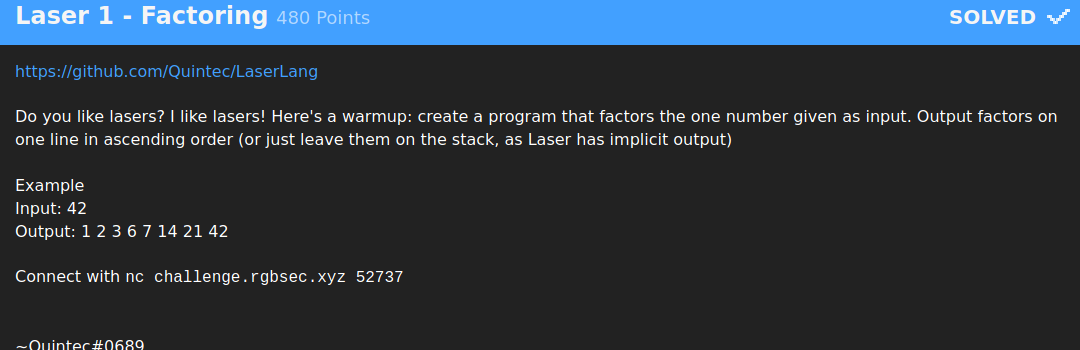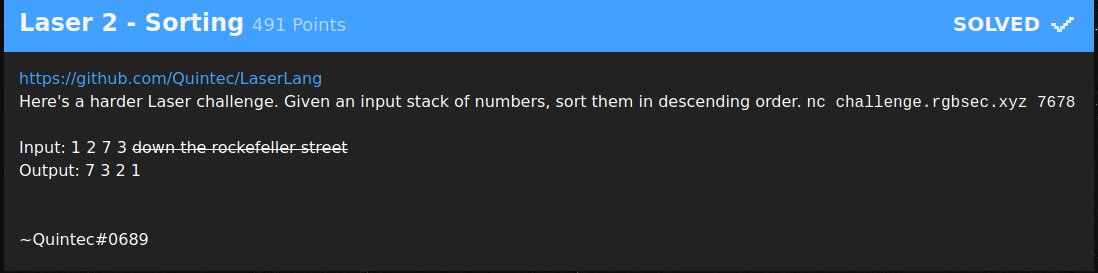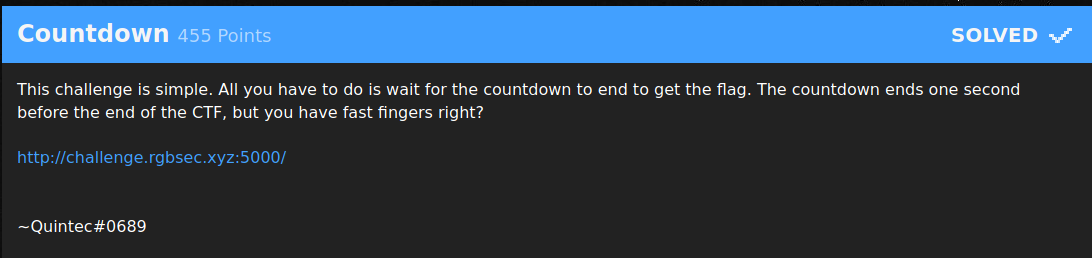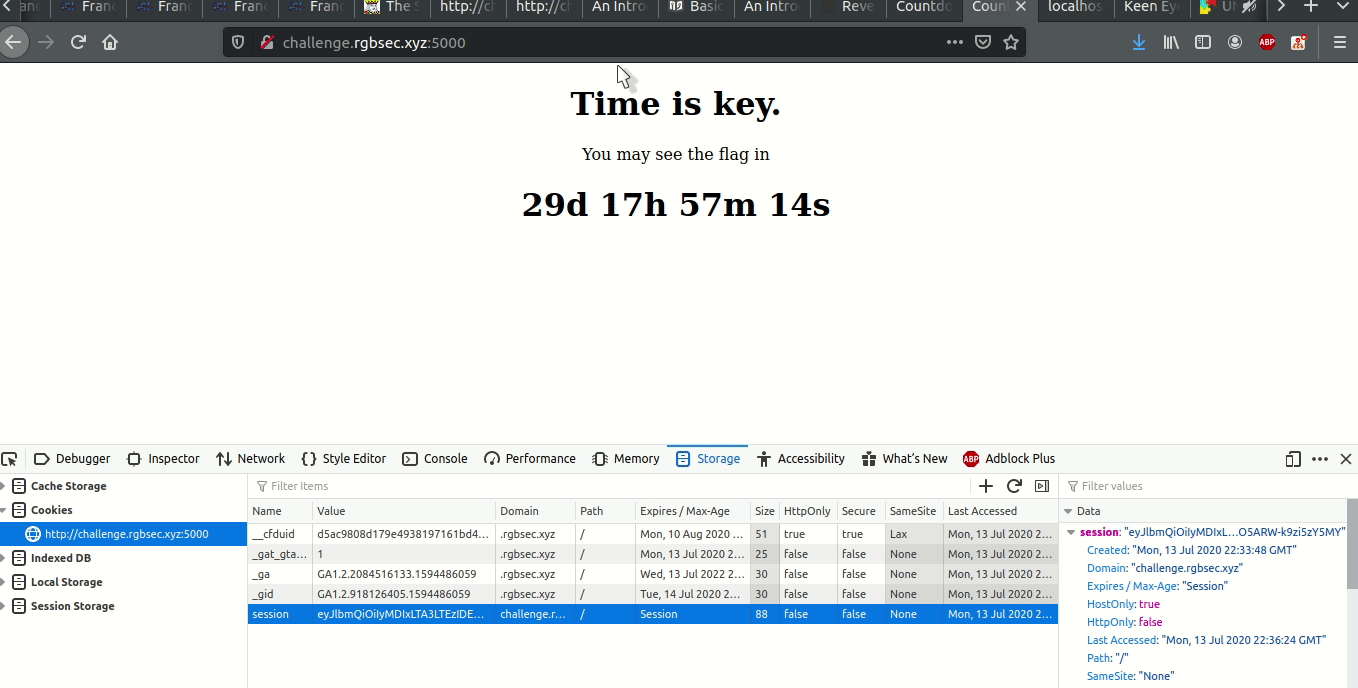# RGBCTF 2020 Writeups

ctf web algo

13 Jul 2020

These are the writeups for the challenges I enjoyed solving during RGBCTF 2020, as a part of the Pwnzorz team. We ended 24th, because unfortunately only another member and I had time to participate this time.

## Laser #

Now I really enjoyed the laser challenges, where you had to implement a trivial algorithm in a difficult language made by the ctf organizers.

Laser is a 2-D language designed to be relatively simple to read code in, even if you have never seen the language before.

Like many 2-D langauges, Laser has an instruction pointer that executes the one character instructions it encounters. The instruction pointer starts at the top left and initially points right. The pointer can wrap around the program and termination only occurs on error or the termination character `#`. The memory structure is a list of stacks. There are only two types in Laser: `String` and `Number`. `Number`s are java `Long`s.

Laser will push any one digit integer it encounters onto the current stack. To input a multi-digit integer, surround the integer with single quotes (`''`). To input a string, surround the string with double quotes (`""`). Note that the instruction pointer will still parse mirrors (next section) as normal when reading strings. To force the interpreter to ignore mirrors, use raw mode and surround your string with backticks (````)

### Laser 1 #

`rgbCTF{l4s3rs_4r3_c00l_r1ght}`So we simply had to list the factors of the input number.

I chose to implement a simple algorithm:

• for all numbers x between 1 and N (inclusive)
• if N % x == 0 - save x on the stack
• Output the stack

We’ll go through the program, in Laser:

``````ir > ruru%  ⌜\
pp
u
r
su
v     ⌜=1r  <<
U  \(p<
#
``````
• Let’s name our stack `S`.
• We begin by pushing the input number onto `S` and then we replicate it with `r`. The last value of our stack is the number, and the one we replicated will be our current iteration of the loop.
• We then have a `>` char that makes sure that whenever we reach the `>` we will be going right - useful for our loop.
• `ruru` is just a set of combinations to duplicate the input and the current iteration so that we can use them in a future operation which is the `modulo`.
• We then check if the result of the modulo is 0 with the `⌜` operator:
• If it is, we `p`op the 0 result of the modulo and then we rotate so we can access the value of the current iteration and `r`eplicate it. We then use `s` which pops the last value on the stack (the current iteration) and pushes it to the above stack which is where we will store our answers.
• Otherwise, we simply pop the result of the modulo.
• We then `r`eplicate the iteration once more and check if we are at iteration 1. If it is, we end the program using the `#` operator which outputs the current stack and ends the program.

### Laser 2 #

`rgbCTF{1_f33l_y0ur_p41n_trust_m3}`So we need to find a way to sort the stack. This post explains a nice way of doing this, by having a tempStack. Check it out for an explanation of the algorithm.

``````>c⌜psUs>c⌜prUrwDg⌜pwv
p      >       >pv
>U#              U
w
^DD                <
^            <
``````

How it works:

• Laser implicitly pushes the input array onto the first stack, but we could use `I` to do it ourselves.
• `c` adds the length of the stack to the stack. We then check if the length of the stack is 0 with `⌜`, and if it is, we end the program and move up to the above stack (`U`) display the contents of the `tmpStack`, which contains our sorted numbers.
• Otherwise, we push `sUs` the last element from the input stack onto a third stack, which will hold that value temporarily.
• We go `U`p to the tempStack and check if it’s empty `c⌜` or not. While it’s not empty and while the last value on the stack of tempStack is bigger than the temp variable on the third stack (`rUrwDg` -> replicate the last value of tempstack and the temp value, compare them and check which is greater), which we just popped from the input stack, we:
• push the last value from `tempStack` onto the input stack
• At the end of this loop, we push the temp variable stored on the third stack onto `tempStack`
• And when the input stack is empty, all we have to do is output `tempStack`

## General Misc #

#### [another witty algo challenge name]#

This is pretty simple. You get a list of 5000 by 5000 grid of ones and zeros, and you have to print the number of islands in the grid.

An island is a collections of ones where each one is adjacent to another one in the island. For a cell to be adjacent to another cell, they must share an edge.

Submit the number wrapped in the flag format, like rgbCTF{123}

So pretty simple, all you needed to do was traverse the input of ones and zeros, and when you cross a one, you use a recursive function that marks the adjacent ones as already being part of an island. Whenever we meet a one that has not been marked this way, we know that it is part of a new island and we call the function on it.

Program:

``````#include <bits/stdc++.h>
using namespace std;
// struct for island positions
struct Position {
bool val, marked = 0;
};
Position island;
// checks if coords are valid
bool isValid (int x, int y)
{
return x < 5000 && x >= 0 && y < 5000 && y >= 0;
}
void checkIsland(int x, int y) {
int dirs = {{-1, 0}, {0, -1}, {1, 0}, {0, 1}};
for(int i = 0; i < 4; i++)
{
int currX = x + dirs[i], currY = y + dirs[i];
// mark all adjacent ones as already being part of an island
if (isValid(currX, currY) && island[currY][currX].val && !island[currY][currX].marked)
{
island[currY][currX].marked = 1;
checkIsland(currX, currY);
}
}
}
int main()
{
for(int y = 0; y < 5000; y++) {
for(int x = 0; x < 5000; x++) {
char val;
cin >> val;
// get boolean input
if (val == '0') island[y][x].val = 0;
else island[y][x].val = 1;
}
}
int nbIslands = 0;
for(int y = 0; y < 5000; y++) {
for(int x = 0; x < 5000; x++)
{
// increment island counter whenever you meet an unmarked one
if (island[y][x].val && !island[y][x].marked)
{
nbIslands++;
checkIsland(x, y);
}
}
}
cout << nbIslands;
}
``````

`rgbctf{119609}`

#### [insert creative algo chall name]#

https://pastebin.com/pKNVLkTs

So this challenge is basically asking you to generate the partitions of a set and to sum the subsets of each result if the partition is of length x. We can add this list of sums to a set, that way we make sure that our valid solutions are unique. And the length of that set will be the result.

``````x = 4
n = 12
r = []

# generate initial list
for i in range(n):
r.append(pow(2, i))

# function that generates all valid partitions
def partition(collection):
if len(collection) == 1:
yield [ collection ]
return

first = collection
for smaller in partition(collection[1:]):
for n, subset in enumerate(smaller):
yield smaller[:n] + [[ first ] + subset]  + smaller[n+1:]
yield [ [ first ] ] + smaller

nbSols = 0
sols = set()
for n, p in enumerate(partition(r), 1):
# check that the partition is valid and matches the constraint that its len is == x
if len(p) == x:
sums = [sum(l) for l in p]
# get the sum of each subset and convert it to a frozenset so we can add it as a unique solution
print(len(sols))
``````

`rgbctf{611501}`

# Web #

I will only be providing a solve for the `Countdown` challenge, because I enjoyed solving it.By looking at the source, we can notice that the server sets a signed `session` cookie that sets the time when the flag will be revealed:

``````	<script type="text/javascript">
var data = cookie.split('.').replace(/_/g, '/').replace(/-/g, '+');
var data = JSON.parse(atob(data));
var end = moment.utc(data['end']);
setInterval(function() {
var dur = moment.duration(end.diff(moment.utc()));
var disp = dur.get("days") + "d " + dur.get("hours") + "h " + dur.get("minutes") + "m " + dur.get("seconds") + "s";
document.getElementById("countdown").innerHTML = disp;
}, 1000);
});
</script>
``````

What we want to do is to create a cookie that, when read by the server, will tell it that the time has passed and it can finally reveal the flag. To do this, we need to figure out how the cookie is signed and reverse-engineer its signature so the server will treat it as valid.

A request header tells us that the server is running:

`Server: Werkzeug/1.0.1 Python/3.6.9`

Flask uses that server software, so we can presume the `session` system is Flask’s.

Flask signs its tokens with a `APPLICATION_KEY` that is set by the developer often encrypted with the HMAC algorithm. The homepage directly tells us that `Time is key.`, a hint that the secret key was actually simply `Time`.

So I ran my own local flask server with the same key and set a session cookie `end` (the same one used by the website) whose value was a timestamp from a past date.

Server code:

``````from flask import Flask, session

app.config['SECRET_KEY'] = 'Time'

@app.route('/')
def index():
session['end'] = "2020-07-13 16:33:59+0000"
return ""

if __name__ == '__main__':
app.run()
``````

All I had to do was copy the cookie, replace the session cookie of the actual website with the one I had gotten, and the server would read this correctly signed session cookie, notice that the `end` event had already passed, and it would give me the flag (I only used pico for a quick edit, I actually use nvim or vscode):Thanks to the organisers, it was really fun!

Subscribe to the blog's Valuable Entropy newsletter: